## OSDM Demo Tutorial – Making of ‘Oh Xee A Moron Demo’

Categories: EDUCATION, OSDM, TUTORIAL
Tags: ,
Published on: September 8, 2014

Hi Folks,
Here is another Oldskool Demomaker Demo Tutorial of my popular production “Oh Xee A Moron, Part 1“.

Also, all elements, such as 3D Objects, Images, Fonts and the Preference .INI Configuration file are also available for download here (OhXeeAMoron.zip)

# Watch Video Capture

To get an idea how the final product looks like, without touching OSDM or downloading anything, watch the video capture that I created below.

Backup URL to Video on vimeo (http://www.vimeo.com/13534545).

Backup Links to Vimeo and YouTube, as well as additional download options (e.G. a Full Screen Version of the Demo) are available at my official demo release page.

# OSDM Settings Screen-By-Screen

## General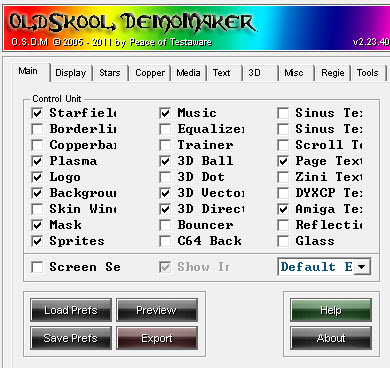Export: Default Export, Enabled Effects : Star Field Music Plasma 3D Ball Page Text Logo Background 3D Vector 3D Direct Amiga Text Mask Sprites

## Display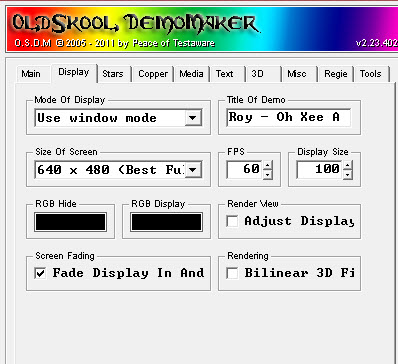Mode: Use Window Mode* (*3D Vectors Positions Will be off, if you select ‘Full Screen’!) Title: Roy – Oh Xee A Moron Size of Screen: 640 x 480 FPS: 60**, Size: 100 (**Timing will be off, if you set it to something else) RGB Hide & RGB Display: Black Render View, Adj Display: False Fade Display In and Out: True Bilinear 3D Filt.: False

## Stars Effect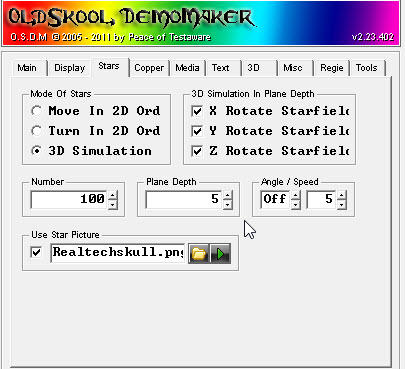Mode: 3D Simulation 3D Sim: X Rot: True, Y Rot: True, Z Rot: True Num: 100, Plane Depth: 5, Angle: Off, Speed: 5 Use Star Picture: True, Image: Realtechskull.png## Copper FX (Plasma)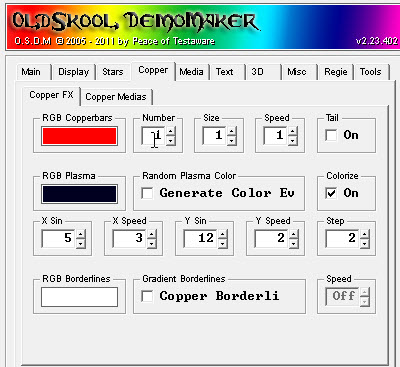RGB Plasma: R=0, G=0, B=32 Random: False, Colorize: True X Sin: 5, X Speed: 3 Y Sin: 12, Y Speed: 2 Step: 2

## Export SettingsIcon: RoySAC.ico
CPU Delay: 1 Start Screen Pic: Roysacny2010-loading2.png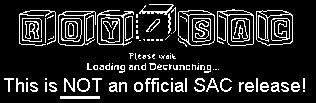Exit by Key: [ESC]

## 3D Direct3D Object: ap-left.jpg Object: Hole, Cull Mode: Render Both Sides X Pos: 320, Y Pos: 240, Z Pos: 4 Camera Angle X/Y/Z: Off X Rot: 1, Y Rot: 1, Z Rot: Off Fog: True, Distance: 2, Intensity: 23 X Scroll: Off, Y Scroll: 1, Z Scroll: Off RGB Hide & RGB Fog: Black (R=0, G=0, B=0)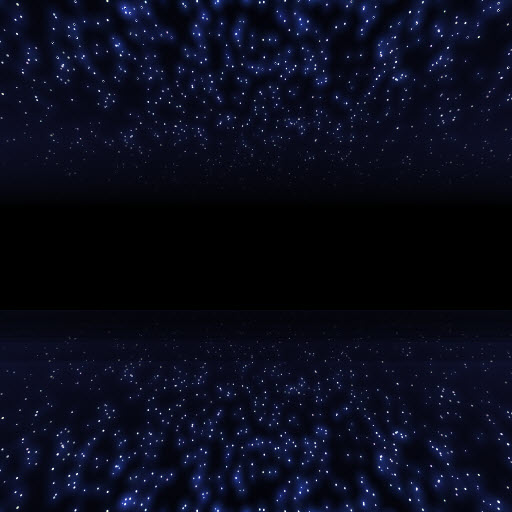## 3D Vector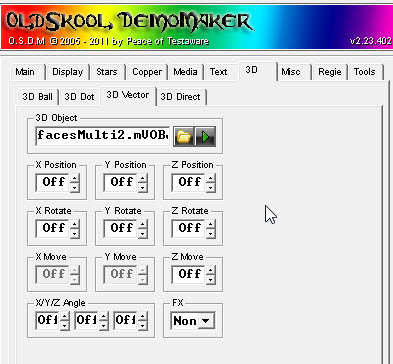3D Object: facesMulti2.mVOBJ Compressed Multi-VOBJ with 5 .VOBJ Objects Settings: Everything = Off

The Objects

 ID=0 (Face) ID=1 (Skull) ID=2 (Hand) ID=3 (Twisty)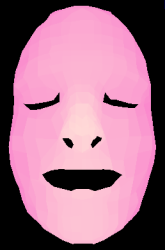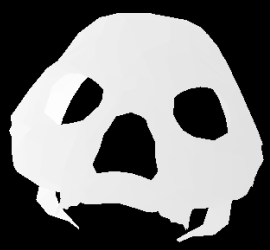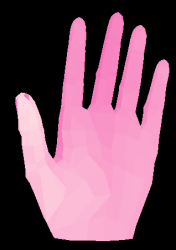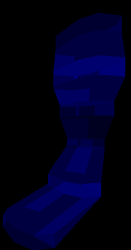ID=4 (RoySAC Logo)### Create Multi-VOBJ Tool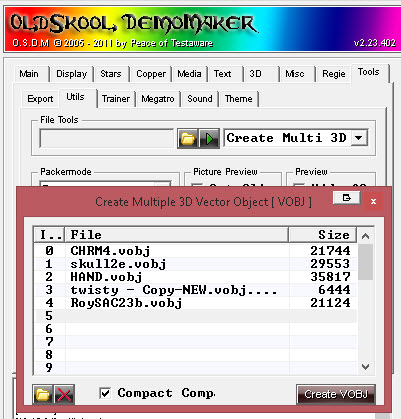Included in the package with all the elements of the demo is already the multi-vector object file (.mVOBJ). But for illustration purposes, I also included the 5 individual (and uncompressed) vector objects (.VOBJ).

To the left is a screen shot of the OSDM build-in tool to merge and compress the objects.

## 3D Ball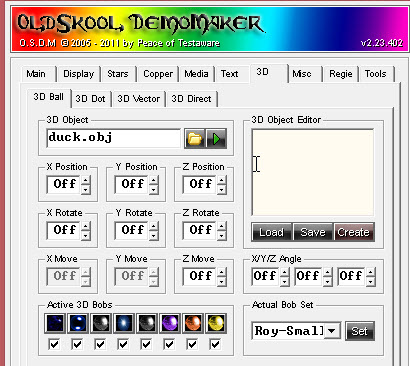• 3D Object: duck.obj
• Everything = Off
• Bobs Balls
 0 1 2 3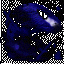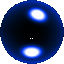4 5 6 7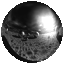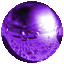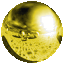## Amiga Text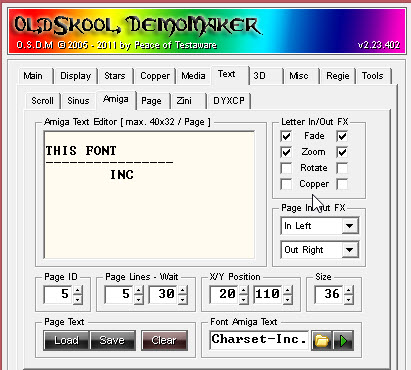Letter In/Out Fx, In & Out: Fade & Zoom Page In: In Left, Page Out: Out Right Page Lines: 5, Wait: 30 X/Y Pos: 20/110, Size: 36 Font: Charset-Inc._stoneage2.pngText (7 Pages): Page 1: ``` DEMO CREDITS ---------------- COMING UP ``` Page 2: ``` CODE ---------------- PEACE - BOBO EPYX ``` Page 3: ``` GFX AND DESIGN ---------------- ROY ``` Page 4: ``` MUSIC "GRIJALBO" ---------------- ESTRAYAK ``` Page 5: ``` THIS FONT ---------------- INC ``` Page 6: ```\$ \$ \$ ``` Page 7: ```\$ \$ \$ \$ ```

## Page Text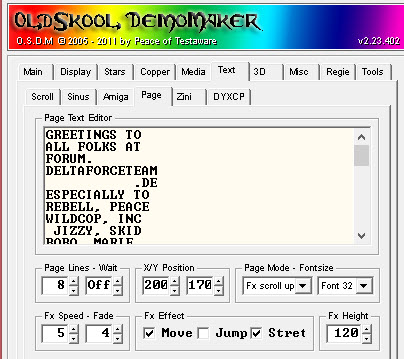Page Lines: 8, Wait: Off, X/Y Pos: 200/170 Mode: Fx Scroll Up, Font-Size: Font 32 Fx Speed: 5, Fade: 4 Fx Effect: Move, Stretch, Fx-Height: 120 Text: ```GREETINGS TO ALL FOLKS AT FORUM. DELTAFORCETEAM .DE ESPECIALLY TO REBELL, PEACE WILDCOP, INC JIZZY, SKID BOBO, MARIE TWILIGHT DRAGON, JANER SYNTH, GENOM NOTE: THIS IS NOT AN OFFICIAL SAC RELEASE, AS ALL OF MY OSDM PRODUCTIONS. CHEERS! CARSTEN AKA ROY OF SAC .... . .. .. .. . . . .. .. . . . .. .. .. . ```

## Logo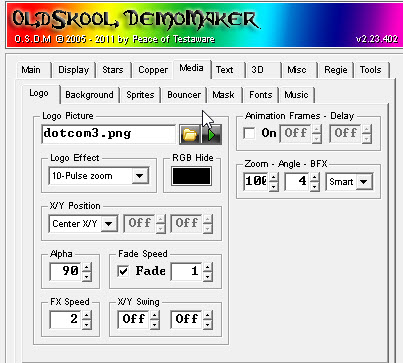Image: dotcom3.png Animation: off Logo Effect: 10 – Pulse Zoom, RGB Hide: Black, Zoom: 100, Angle: 4, BFX: Smart X/Y Position: Center X/Y Alpha: 90, Fade: True, Speed: 1 FX Speed: 2, X/Y Swing: Off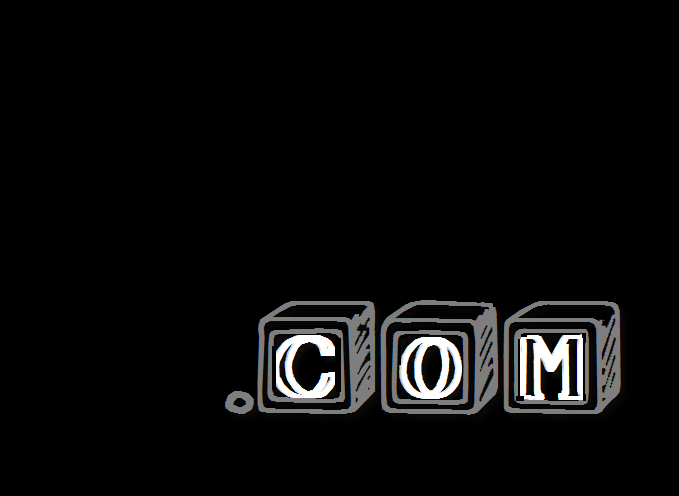## Backgroundimage: starbacknew.jpg Horizontal Scroll: None Vertical Scroll: 4 – Swing Up Mirror: True Zoom: 3 – Horizontal Size## Sprites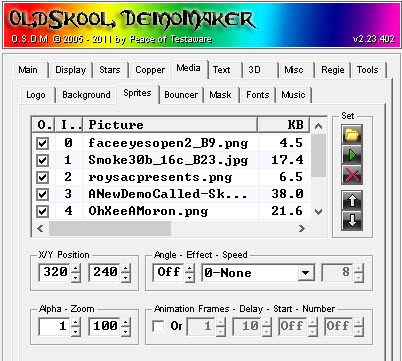Initial Sprites Settings

# Name X/Y-Pos Ang Eff Speed Alpha Zoom Anim Frms Delay Start Num
0 faceeyesopen2_B9.png 320/240 Off 100 100 True 9 80 Off Off
1 Smoke30b_16c_B23.jpg 320/240 Off 30 710 True 23 35 Off Off
2 roysacpresents.png 320/240 13 1-Rotate_Left 1 100 45 False
3 ANewDemoCalled-Skulls.png 320/240 Off 100 100 False
4 OhXeeAMoron.png 320/240 340 2-Rotate_Right 1 100 100 False
5 visitroysaccom.png 320/240 Off 1 100 False
6 fuckingvisit.png 320/240 320 2-Rotate_Right 1 100 100 False
 0 – faceeyesopen2_B9.png 6 – fuckingvisit.png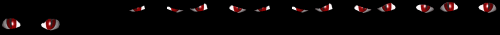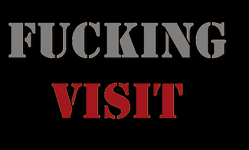1 – Smoke30b_16c_B23.jpg 7 – RadialGradientBG.png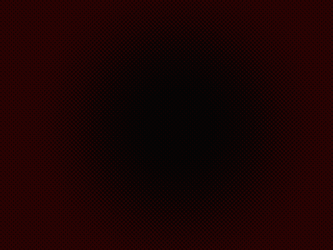2 – roysacpresents.png 3 – ANewDemoCalled-Skulls.png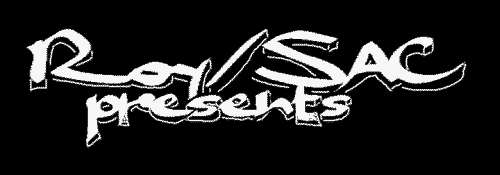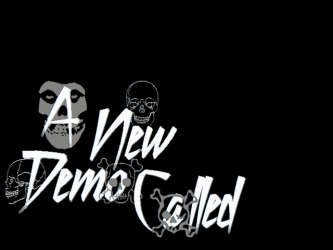4 – OhXeeAMoron.png 5 – visitroysaccom.png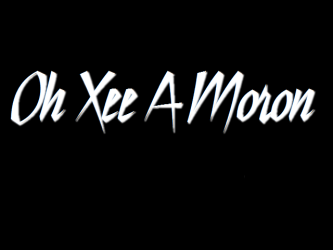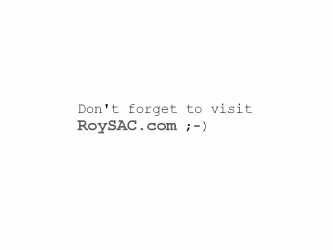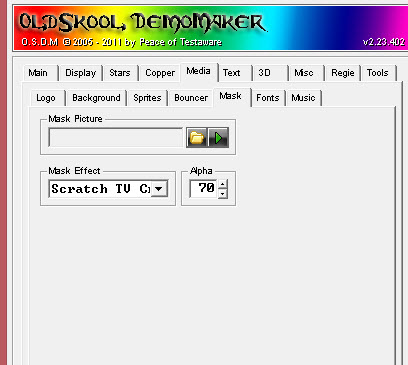Mask Effect: Scratch TV Crumble, Alpha: 70

## Fonts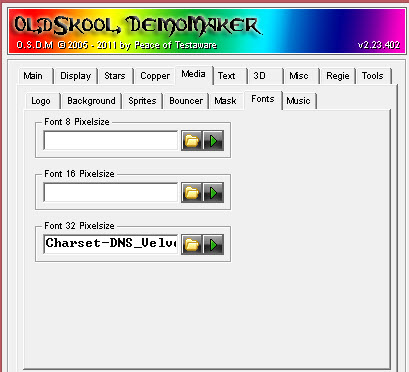Font 32 Pixelsize: Charset-DNS_Velvenda Cooler Font.png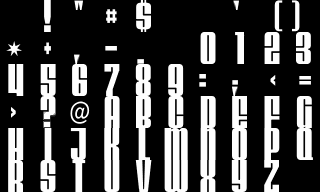## Music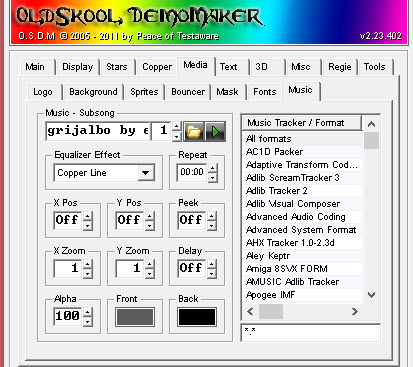File: grijalbo by estrayak-modified.mod, Track: 1

## Script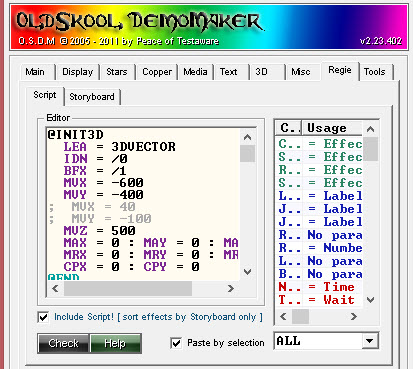Make sure that “include Script! [sort effects by Storyboard only]” is Checked See Script Code below

## Storyboard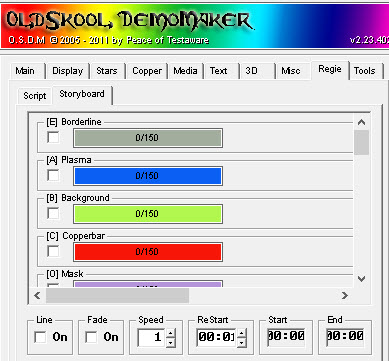Only Enabled Effects are listed. All the others don’t matter. Initial Effects Order [A] Plasma [B] Background [O] Mask [D] Starfield [Q] 3D Direct Object [G] 3D Vector Object [J] Logo [I] 3D Ball Object [N] Page Text [W] Amiga Text [X] Sprite

# The Script Code

```@INIT3D
LEA = 3DVECTOR
IDN = /0 : BFX = /1 : MVX = -600 : MVY = -400
;  MVX = 40 : MVY = -100
MVZ = 500
MAX = 0 : MAY = 0 : MAZ = 0
MRX = 0 : MRY = 0 : MRZ = 0
CPX = 0 : CPY = 0
@END
@INI3DBA
LEA = 3DBALL
;  IDN = /0
BFX = /1 : MVX = -500 : MVY = -200
;  MVX = 0 : MVY = 0
MVZ = 500
@END

FPS = 60
CAL = MUSIC
JSR = 1
JSR = 10
JSR = 20
JSR = 30
JSR = 40
JSR = 50
JSR = 60

CAL = SPRITE : LEA = SPRITE
IDN = 5 : MVA = 255
CAL = DISPLAY
NOP = 1
REP = 99 : ADV = -1 : LOP
NOP = 1
ESC : END

;-----------
LAB = 1
CAL = SPRITE : LEA = SPRITE
IDN = 0 : MVA = 0
IDL = 3DVECTOR
IDN = 2 : MVA = 0
IDN = 3 : MVA = 0
IDN = 4 : MVA = 0
IDN = 5 : MVA = 0
IDN = 6 : MVA = 0
IDN = 7 : MVA = 0
STP = SPRITE
RTS
;---------------
LAB = 10
CAL = SPRITE+3DVECTOR
@INIT3D = 0,2
REP = 135 : ADZ = -10 : LOP
MRY = 6
LEA = SPRITE : IDN = 1
REP = 100 : ADH = 2 : LOP
LEA = 3DVECTOR : IDN = 0
NOP = 1 : MRY = -6
NOP = 4 : MRY = 6
LEA = SPRITE : IDN = 1
REP = 75 : ADY = -1 : ADH = -1 : ADW = -1 : LOP
LEA = 3DVECTOR : IDN = 0
NOP = 1 : MRY = 0
LEA = SPRITE : IDN = 1
REP = 54 : ADA = -4 : LOP
MVA = 0
IDL = 3DVECTOR : IDN = 0
NOP = 2
LEA = SPRITE : IDN = 0 : MVA = 0
LEA = 3DVECTOR : IDN = 0
REP = 50 : ADZ = 80 : ADY = 2 : LOP
RGB = \$F00 :CLS = 2
STP = 3DVECTOR+SPRITE
RTS
;---------------
LAB = 20
CAL = 3DVECTOR
@INIT3D = 1,1
ADZ = 600 : MAX = 90 : MAY = -70 : ADY = 120
REP = 50 : ADZ = -12 : ADY = -2 : LOP
MRY = 3
NOP = 3 : MRY = -3
REP = 50 : ADX = -2 : ADZ = -1 : LOP
REP = 300 : ADX = 1 : ADZ = 3 : LOP
BFX = 4
REP = 100 : ADX = -1 : ADZ = -4 : LOP
CAL = SPRITE : LEA = SPRITE : IDN = 2
REP = 54 : ADA = 4 : LOP
MVA = 255
CAL = MASK : NOP = 1
LEA = 3DVECTOR : IDN = 1
MRY = 3
REP = 75 : ADZ = -5 : LOP
LEA = SPRITE
REP = 54
IDN = 2 : ADA = -4
IDN = 3 : ADA = 4
LOP
IDN = 2 : MVA = 0
IDN = 3 : MVA = 255
NOP = 2
CAL = MASK : NOP = 2
LEA = 3DVECTOR : IDN = 1
MRY = -3
REP = 100 : ADZ = 20 : ADX = -1 : LOP
STP = MASK : LEA = SPRITE
REP = 54
IDN = 3 : ADA = -4
IDN = 4 : ADA = 4
LOP
IDN = 3 : MVA = 0
IDN = 4 : MVA = 255
NOP = 1
CAL = MASK : NOP = 1
LEA = 3DVECTOR : IDN = 1
REP = 50 : ADZ = 20 : LOP
LEA = SPRITE : IDN = 4
REP = 54 : ADA = -4 : LOP
MVA = 0 : CLS = 2
RTS

;--------------------
LAB = 30
CAL = 3DDIRECT
@INIT3D = 2,2
AAY = 90 : AAX = 10 : AAZ = 20
MRY = -6 : MRX = 6 : MRZ = 8
REP = 100
AAX = 1 : ADZ = -20 : ADY = 4 : AAX = -1
LOP
REP = 100
LOP
REP = 100
LOP
NOP = 1
REP = 100
LOP
NOP = 5
REP = 300 : ADY = -4 : LOP
RGB = \$100
STP = 3DVECTOR
LEA = SPRITE : IDN = 4 : MVA = 0
STP = SPRITE
RTS

;----------------
LAB = 40
CAL = BACK
LEA = 3DDIRECT
REP = 150 : ADZ = -8 : LOP
STP = 3DDIRECT : NOP = 1
CLS = 3
CAL = 3DVECTOR
@INIT3D = 3,4
CPH = 320 : CPY = 20
CAL = AMIGATEXT
LEA = 3DVECTOR : IDN = 3
REP = 1500 : ADY = -3 : LOP
REP = 200 : ADX = 3 : LOP
STP = 3DVECTOR+DISPLAY
NOP = 1
STP = AMIGATEXT+BACK
RTS

;-----------------
LAB = 50
CAL = PLASMA+3DBALL
@INI3DBA = 0,2
MRX = 15 : MRY = 15 : MRZ = 15
CAL = DISPLAY+PAGE
REP = 200 : ADX = 2 : LOP
REP = 125 : ADZ = 1 : LOP
CAL = MASK : BFX = 1
REP = 125 : ADZ = 1 : LOP
STP = MASK : BFX = 2
REP = 250 : ADZ = 1 : LOP
CAL = MASK : BFX = 3
REP = 150 : ADZ = -1 : LOP
STP = MASK : BFX = 4
REP = 150 : ADZ = -1 : LOP
REP = 150 : ADZ = -1 : LOP
CAL = MASK : BFX = 1
REP = 150 : ADZ = -1 : LOP
STP = MASK : BFX = 4
REP = 100 : ADX = 2 : LOP
STP = DISPLAY : NOP = 1
STP = PAGE+3DBALL+PLASMA
RTS

;-----------------
LAB = 60
CAL = STARS+PLASMA+SPRITE
LEA = SPRITE
IDN = 6 : MVA = 0
IDN = 1
IDL = 3DVECTOR
SFX = 2 : BFX = 2
CAL = 3DVECTOR
@INIT3D = 4,4
IDL = 3DVECTOR : LEA = 3DVECTOR : IDN = 4
REP = 180
AAX = 2 : ADZ = -10
IFR > 8 : ADY = 1
LOP
AAX = -360
REP = 184
AAX = 2 : ADZ = -10
IFR > 8 : ADY = 1
LOP
MRX = 0 : MRY = 25 : BFX = 4
CAL = LOGO
LEA = 3DVECTOR : IDN = 4
IDL = SPRITE
REP = 100 : ADY =  -1 : LOP
LEA = LOGO
REP = 50
LEA = LOGO
LEA = SPRITE
LOP
LEA = SPRITE : IDN = 1 : MVA = 0
LEA = LOGO
IDL = 3DVECTOR
LEA = SPRITE : IDN = 6 : MVX = 200 : MVY = 150
REP = 54
LOP
IDL = TRAINER : IDN = 7
REP = 50 : ADA = 2 : LOP
NOP = 4
LEA = SPRITE : IDN = 6
REP = 40 : ADX = -2 : LOP
REP = 100 : ADX = 2 : ADA = 2 : LOP
REP = 100 : ADX = 2 : ADA = -2 : LOP
MVA = 0
CAL = MASK : LEA = LOGO
REP = 50 : ADX = -2 : ADY = -1 : LOP
REP = 200 : ADX = 2 : LOP
REP = 100 : ADX = -2 : LOP
REP = 125 : AAX = 1 : ADH = -2 : ADW = 2 : ADA = -2 : ADY = 2
LOP
STP = LOGO
LEA = 3DVECTOR : IDN = 4
REP = 200 : ADY = 2 : LOP
STP = 3DVECTOR+DISPLAY
NOP = 1
STP = STARS
LEA = SPRITE : IDN = 7 : MVA = 0
RTS
```

# Finalizing

For all of my OSDM productions, tools by me and others, Oldskool Demomaker resources and communities, check out the OSDM Section of my web site.

Enjoy!

Cheers!
Carsten aka Roy/SAC

NOTE! I believe in the right for freedom of speech and personal opinion and are against censorship, so feel free to tell me what you think and let me and others hear your opinion on this subject, but please avoid using the f-word and s-word as much as you possibly can, because at the end of the day this blog exists for the purpose of useful exchanges of thoughts, ideas and opinions and not as a valve for your accumulated anger and frustration. Get a shrink for that! Thanks.

Welcome , today is Wednesday, October 20, 2021